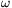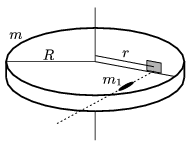Mathematical and Physical Journal
for High Schools
Issued by the MATFUND Foundation
 Already signed up? New to KöMaL?

#Problem P. 3898. (May 2006)

P. 3898. A horizontal disc of mass m=0,6 kg and radius R=10 cm can be rotated easily about a vertical axle. Along a radius of the disc a sheet is fixed to the disc. (The mass of the sheet is negligible.) A horizontally fired bullet, whose mass is m1=20 g, hits the sheet perpendicularly, and the disc begins to rotate at an angular velocity of=20 s-1. The distance between the axle and the place of impact is r=8 cm. What was the initial speed of the bullet, if a) it is embedded in the sheet; b) it bounced back totally elastically?(5 pont)

Deadline expired on June 12, 2006.

### Statistics:

 81 students sent a solution. 5 points: 52 students. 4 points: 15 students. 3 points: 11 students. 2 points: 2 students. 0 point: 1 student.

Problems in Physics of KöMaL, May 2006